• No products in the cart.

# 204.5.12 Practice : Digit Recognizer

##### Implementing Neural Network on digit image data.

Link to the previous post : https://statinfer.com/204-5-11-hidden-layers-and-their-roles/

As promised, in the first post of the series we will build a Neural Network that will read the image of a digit and correctly identify the number.

## Practice : Digit Recognizer

• Take an image of a handwritten single digit, and determine what that digit is.
• Normalized handwritten digits, automatically scanned from envelopes by the U.S. Postal Service. The original scanned digits are binary and of different sizes and orientations; the images here have been de slanted and size normalized, resultingin 16 x 16 grayscale images (Le Cun et al., 1990).
• The data are in two gzipped files, and each line consists of the digitid (0-9) followed by the 256 grayscale values.
• Build a neural network model that can be used as the digit recognizer.
• Use the test dataset to validate the true classification power of the model
• What is the final accuracy of the model?
• We can see them as multiple lines on the decision spaceIn :
#Importing test and training data
import numpy as np

In :
#digits_train is numpy array. we convert it into dataframe for better handling
train_data=pd.DataFrame(digits_train)
train_data.shape

Out:
(7291, 257)
In :
digits_test = np.loadtxt("datasets\\Digit Recognizer\\USPS\\zip.test.txt")
#digits_test is numpy array. we convert it into dataframe for better handling
test_data=pd.DataFrame(digits_test)
test_data.shape

Out:
(2007, 257)
In :
train_data.value_counts()     #To get labels of the images

Out:
0.0    1194
1.0    1005
2.0     731
6.0     664
3.0     658
4.0     652
7.0     645
9.0     644
5.0     556
8.0     542
Name: 0, dtype: int64
In :
import matplotlib.pyplot as plt

#Lets have a look at some images.

for i in range(0,5):
data_row=digits_train[i][1:]
#pixels = matrix(as.numeric(data_row),16,16,byrow=TRUE)
pixels = np.matrix(data_row)
pixels=pixels.reshape(16,16)
plt.figure(figsize=(10,10))
plt.subplot(3,3,i+1)
plt.imshow(pixels)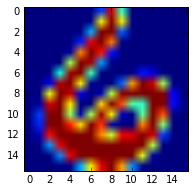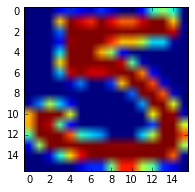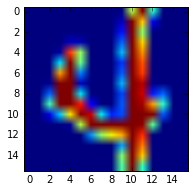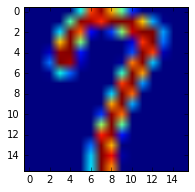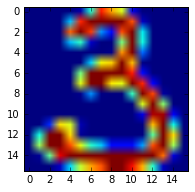In :
#Creating multiple columns for multiple outputs
#####We need these variables while building the model
digit_labels=pd.DataFrame()
digit_labels['label']=train_data[0:]
label_names=['I0','I1','I2','I3','I4','I5','I6','I7','I8','I9']
for i in range(0,10):
digit_labels[label_names[i]]=digit_labels.label==i
#see our newly created labels data

Out:
label I0 I1 I2 I3 I4 I5 I6 I7 I8 I9
0 6.0 False False False False False False True False False False
1 5.0 False False False False False True False False False False
2 4.0 False False False False True False False False False False
3 7.0 False False False False False False False True False False
4 3.0 False False False True False False False False False False
5 6.0 False False False False False False True False False False
6 3.0 False False False True False False False False False False
7 1.0 False True False False False False False False False False
8 0.0 True False False False False False False False False False
9 1.0 False True False False False False False False False False
In :
#Update the training dataset
train_data1=pd.concat([train_data,digit_labels],axis=1)
print(train_data1.shape)

(7291, 268)

Out:
0 1 2 3 4 5 6 7 8 9 I0 I1 I2 I3 I4 I5 I6 I7 I8 I9
0 6.0 -1.0 -1.0 -1.0 -1.000 -1.000 -1.000 -1.000 -0.631 0.862 False False False False False False True False False False
1 5.0 -1.0 -1.0 -1.0 -0.813 -0.671 -0.809 -0.887 -0.671 -0.853 False False False False False True False False False False
2 4.0 -1.0 -1.0 -1.0 -1.000 -1.000 -1.000 -1.000 -1.000 -1.000 False False False False True False False False False False
3 7.0 -1.0 -1.0 -1.0 -1.000 -1.000 -0.273 0.684 0.960 0.450 False False False False False False False True False False
4 3.0 -1.0 -1.0 -1.0 -1.000 -1.000 -0.928 -0.204 0.751 0.466 False False False True False False False False False False

5 rows × 268 columns

In :
#########Neural network building
import neurolab as nl
import numpy as np
import pylab as pl

x_train=train_data.drop(train_data.columns[], axis=1)
y_train=digit_labels.drop(digit_labels.columns[], axis=1)

In :
#getting minimum and maximum of each column of x_train into a list
def minMax(x):
return pd.Series(index=['min','max'],data=[x.min(),x.max()])

In :
listvalues = x_train.apply(minMax).T.values.tolist()

error = []

In :
# Create network with 1 layer and random initialized
net = nl.net.newff(listvalues,[20,10],transf=[nl.trans.LogSig()] * 2)
net.trainf = nl.train.train_rprop

In :
# Train network
import time
start_time = time.time()
error.append(net.train(x_train, y_train, show=0, epochs = 250,goal=0.02))
print("--- %s seconds ---" % (time.time() - start_time))

--- 286.51438784599304 seconds ---

In :
# Prediction testing data
x_test=test_data.drop(test_data.columns[], axis=1)
y_test=test_data[0:]

predicted_values = net.sim(x_test.as_matrix())
predict=pd.DataFrame(predicted_values)

index=predict.idxmax(axis=1)

In :
#confusion matrix
from sklearn.metrics import confusion_matrix as cm
ConfusionMatrix = cm(y_test,index)
print('Confusion Matrix : ', ConfusionMatrix)

#accuracy
accuracy=np.trace(ConfusionMatrix)/sum(sum(ConfusionMatrix))
print('Accuracy : ', accuracy)

error=1-accuracy
print('Error : ', error)

Confusion Matrix :  [[339   0   5   3   2   4   5   1   0   0]
[  0 249   2   2   3   1   4   1   1   1]
[  4   0 169   4   7   2   6   1   5   0]
[  3   0   5 143   0   9   0   1   2   3]
[  0   2   4   0 180   4   2   2   1   5]
[  6   0   2  10   2 134   0   2   1   3]
[  4   0   3   0   4   5 152   0   2   0]
[  0   0   1   1   4   0   0 135   2   4]
[  6   1   2   7   1   5   1   3 137   3]
[  0   1   1   0   2   1   0   3   1 168]]
Accuracy :  0.899850523169
Error :  0.100149476831

The next post is a conclusion on neural network.
Link to the next post : https://statinfer.com/204-5-13-neural-networks-conclusion/

### 0 responses on "204.5.12 Practice : Digit Recognizer"

Statinfer Software Solutions LLP

Software Technology Parks of India,
NH16, Krishna Nagar, Benz Circle,